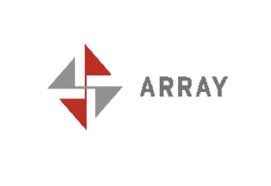3. Can the sizeof operator be used to tell the size of an array passed to a function?

No. There’s no way to tell, at runtime, how many elements are in an array parameter just by looking at the array parameter itself. Remember, passing an array to a function is exactly the same as passing a pointer to the first element. This is a Good Thing. It means that passing pointers and arrays to C functions is very efficient.
It also means that the programmer must use some mechanism to tell how big such an array is. There are two common ways to do that. The first method is to pass a count along with the array. This is what memcpy() does, for example:

char    source[ MAX ], dest[ MAX ];

/* … */

memcpy( dest, source, MAX );

The second method is to have some convention about when the array ends. For example, a C “string” is just a pointer to the first character; the string is terminated by an ASCII NUL (‘\0′) character. This is also commonly done when you have an array of pointers; the last is the null pointer. Consider the following function, which takes an array of char*s. The last char* in the array is NULL; that’s how the function knows when to stop.

void printMany( char *strings[] )

{

int     i;

i = 0;

while ( strings[ i ] != NULL )

{

puts( strings[ i ] );

++i;

}

}

Most C programmers would write this code a little more cryptically:

void  printMany( char *strings[] )

{

while ( *strings )

{

puts( *strings++ );

}

}

C programmers often use pointers rather than indices. You can’t change the value of an array tag, but because strings is an array parameter, it’s really the same as a pointer. That’s why you can increment strings. Also,

while ( *strings )

means the same thing as

while ( *strings != NULL )

and the increment can be moved up into the call to puts().

4. Is it better to use a pointer to navigate an array of values, or is it better to use a subscripted array name?

It’s easier for a C compiler to generate good code for pointers than for subscripts.

Say that you have this:

/* X is some type */

X       a[ MAX ];       /* array */

X       *p;     /* pointer */

X       x;      /* element */

int     i;      /* index */

Here’s one way to loop through all elements:

/* version (a) */

for ( i = 0; i < MAX; ++i )

{

x = a[ i ];

/* do something with x */

}

On the other hand, you could write the loop this way:

/* version (b) */

for ( p = a; p < & a[ MAX ]; ++p )

{

x = *p;

/* do something with x */

}

T
The pointer version makes the comparison simpler:

/* version (d) */

for ( p = & a[ MAX – 1 ]; p >= a; –p )

{

x = *p;

/* do something with x */

}